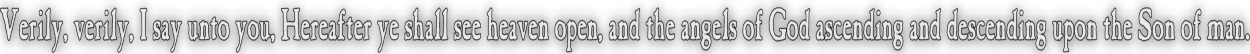None:
Polyps:
Strongs:MetaphysicsUltraproduct - The Sowing Of The Word An ultraproduct is an (possibly) infinite dimensional cartestian product forming an indexing set on the components under an ultrafilter U. The product is formed all of factors of a structure M. Then the operation upon the set of each factor is naturally taken to be as taken componentwise that in two sequences A and B, we have (A+B)i = Ai + Bi Then, we may state that any two sequences in the product are "equal almost everywhere" if the set of all 'i' (coordinates) where Ai = Bi is in the ultrafilter U. Then, we may place each "equal" set of sequences into classes and act upon them with the componentwise product - we call this new structure an "ultraproduct". NB: with a principal ultrafilter we simply return an ultraproduct isomorphic to the factor at the coordinate corresponding to the principal element in the ultrafilter. We will start our product with each factor M equal to the set of all positive properties inclusive of virtues. And we will state that two sequences formed from our product in the Mi accumulate sets of positive properties under inclusion as the coordinates are increasing, So that the sequence in M x M x M x,.. = (a,b,c,d,e,...) is actually made into the sequence ({a},{a,b},{a,b,c},{a,b,c,d},{a,b,c,d,e},...) Then, we state that two sequences are equal if and only if they both contain L(G) at the same coordinate. As we require that L(G) absorb virtues and suppress G v p statements, we use L(G) to "reset the count" (to {L(G)} alone) when under inclusion. Then of our four cases in the parable of the sower, we must also equate the sequences that contian L(q) or L(p) as if they were L(X). Since these represent arbitrary sets of positive properties, we must assume there is some limiting assumption when L(G) was "sown" i.e. our initial assumptions "x". So we may state that any two sequences are equal at a coordinate if they contain L(G) & x, where 'x' is the same set of minimal expectations of God. (We would reset the count to L(G) , as if we were given "x".) We would call the result L(X). Then under sequence (i),(ii),(iii) we have a unique descriptor for our sequences, (as we assume we have to start L(X) = L(G \ L(G)) for sequences of type (iii).) The result is that sequences will tend to L(G) or diverge from it. Type (ii) is such that L(G)&q tends to itself, and type (i) to itself also. Then if every coordinate in our sum contains one positive property it must be L(G) or at least some L(X). That way, the word is sown as it is heard. We keep the "essence" L(G) of all such L(X) to be intrinsic to the definition of L(X), i.e. L(X) = L(G)&x. Therefore we have under inclusion if we make our ultrafilter non-principal, we have sequences that terminate in L(G), or ¬L(G) or some other L(X). L(G) becomes the conjunction of every positive property expected of God. If a sequence under inclusion tends to "{L(G)} only" (type (iv)) at almost every coordinate, then we may safely state that that sequence is positive, and likewise every other sequence tending elsewhere to some other L(X) to be negative. Of course, we assert pos(G~x) in the sense of the former! Then if we may divide into equivalence classes we have all those holding L(G) and all those with each L(X) - each class corresponding to holding some L(X) true "almost everywhere". Alternatively we have the fruitful L(G) and the unfruitful L(X). There are many more X's than the one "G",.. So we have many called but few chosen. Continue To Next Page Return To Section Start Return To Previous Page'